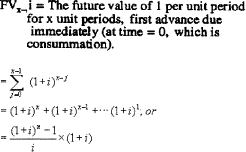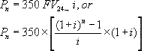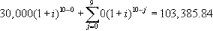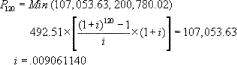An official website of the United States government

# Appendix K to Part 1026 — Total Annual Loan Cost Rate Computations for Reverse Mortgage Transactions

This version is not the current regulation.

You are viewing a previous version of this regulation with amendments that went into effect on Feb. 17, 2021. View all versions of this regulation

1. The calculation of total annual loan cost rates under appendix K is based on the principles set forth and the estimation or “iteration” procedure used to compute annual percentage rates under appendix J. Rather than restate this iteration process in full, the regulation cross-references the procedures found in appendix J. In other aspects the appendix reflects the special nature of reverse mortgage transactions. Special definitions and instructions are included where appropriate.

(b) Instructions and equations for the total annual loan cost rate

(b)(5) Number of unit-periods between two given dates

1. Assumption as to when transaction begins. The computation of the total annual loan cost rate is based on the assumption that the reverse mortgage transaction begins on the first day of the month in which consummation is estimated to occur. Therefore, fractional unit-periods (used under appendix J for calculating annual percentage rates) are not used.

(b)(9) Assumption for discretionary cash advances

1. Amount of credit. Creditors should compute the total annual loan cost rates for transactions involving discretionary cash advances by assuming that 50 percent of the initial amount of the credit available under the transaction is advanced at closing or, in an open-end transaction, when the consumer becomes obligated under the plan. (For the purposes of this assumption, the initial amount of the credit is the principal loan amount less any costs to the consumer under § 1026.33(c)(1).)

(b)(10) Assumption for variable-rate reverse mortgage transactions

1. Initial discount or premium rate. Where a variable-rate reverse mortgage transaction includes an initial discount or premium rate, the creditor should apply the same rules for calculating the total annual loan cost rate as are applied when calculating the annual percentage rate for a loan with an initial discount or premium rate (see the commentary to § 1026.17(c)).

(d) Reverse mortgage model form and sample form

(d)(2) Sample form

1. General. The “clear and conspicuous” standard for reverse mortgage disclosures does not require disclosures to be printed in any particular type size. Disclosures may be made on more than one page, and use both the front and the reverse sides, as long as the pages constitute an integrated document and the table disclosing the total annual loan cost rates is on a single page.

See interpretation of General. in Supplement I

(a) Introduction. Creditors are required to disclose a series of total annual loan cost rates for each reverse mortgage transaction. This appendix contains the equations creditors must use in computing the total annual loan cost rate for various transactions, as well as instructions, explanations, and examples for various transactions. This appendix is modeled after appendix J of this part (Annual Percentage Rates Computations for Closed-end Credit Transactions); creditors should consult appendix J of this part for additional guidance in using the formulas for reverse mortgages.

(b) Instructions and equations for the total annual loan cost rate

(1) General rule. The total annual loan cost rate shall be the nominal total annual loan cost rate determined by multiplying the unit-period rate by the number of unit-periods in a year.

(2) Term of the transaction. For purposes of total annual loan cost disclosures, the term of a reverse mortgage transaction is assumed to begin on the first of the month in which consummation is expected to occur. If a loan cost or any portion of a loan cost is initially incurred beginning on a date later than consummation, the term of the transaction is assumed to begin on the first of the month in which that loan cost is incurred. For purposes of total annual loan cost disclosures, the term ends on each of the assumed loan periods specified in § 1026.33(c)(6).

(3) Definitions of time intervals.

(i) A period is the interval of time between advances.

(ii) A common period is any period that occurs more than once in a transaction.

(iii) A standard interval of time is a day, week, semimonth, month, or a multiple of a week or a month up to, but not exceeding, 1 year.

(iv) All months shall be considered to have an equal number of days.

(4) Unit-period.

(i) In all transactions other than single-advance, single-payment transactions, the unit-period shall be that common period, not to exceed one year, that occurs most frequently in the transaction, except that:

(A) If two or more common periods occur with equal frequency, the smaller of such common periods shall be the unit-period; or

(B) If there is no common period in the transaction, the unit-period shall be that period which is the average of all periods rounded to the nearest whole standard interval of time. If the average is equally near two standard intervals of time, the lower shall be the unit-period.

(ii) In a single-advance, single-payment transaction, the unit-period shall be the term of the transaction, but shall not exceed one year.

(5) Number of unit-periods between two given dates.

(i) The number of days between two dates shall be the number of 24-hour intervals between any point in time on the first date to the same point in time on the second date.

(ii) If the unit-period is a month, the number of full unit-periods between two dates shall be the number of months. If the unit-period is a month, the number of unit-periods per year shall be 12.

(iii) If the unit-period is a semimonth or a multiple of a month not exceeding 11 months, the number of days between two dates shall be 30 times the number of full months. The number of full unit-periods shall be determined by dividing the number of days by 15 in the case of a semimonthly unit-period or by the appropriate multiple of 30 in the case of a multimonthly unit-period. If the unit-period is a semimonth, the number of unit-periods per year shall be 24. If the number of unit-periods is a multiple of a month, the number of unit-periods per year shall be 12 divided by the number of months per unit-period.

(iv) If the unit-period is a day, a week, or a multiple of a week, the number of full unit-periods shall be determined by dividing the number of days between the two given dates by the number of days per unit-period. If the unit-period is a day, the number of unit-periods per year shall be 365. If the unit-period is a week or a multiple of a week, the number of unit-periods per year shall be 52 divided by the number of weeks per unit-period.

(v) If the unit-period is a year, the number of full unit-periods between two dates shall be the number of full years (each equal to 12 months).

(6) Symbols. The symbols used to express the terms of a transaction in the equation set forth in paragraph (b)(8) of this appendix are defined as follows:

Aj = The amount of each periodic or lump-sum advance to the consumer under the reverse mortgage transaction.

i = Percentage rate of the total annual loan cost per unit-period, expressed as a decimal equivalent.

j = The number of unit-periods until the jth advance.

n = The number of unit-periods between consummation and repayment of the debt.

Pn = Min (Baln, Valn). This is the maximum amount that the creditor can be repaid at the specified loan term.

Baln = Loan balance at time of repayment, including all costs and fees incurred by the consumer (including any shared appreciation or shared equity amount) compounded to time n at the creditor's contract rate of interest.

Valn = Val0(1 + σ)

σ = The summation operator.

Symbols used in the examples shown in this appendix are defined as follows:w = The number of unit-periods per year.

I = wi × 100 = the nominal total annual loan cost rate.

(7) General equation. The total annual loan cost rate for a reverse mortgage transaction must be determined by first solving the following formula, which sets forth the relationship between the advances to the consumer and the amount owed to the creditor under the terms of the reverse mortgage agreement for the loan cost rate per unit-period (the loan cost rate per unit-period is then multiplied by the number of unit-periods per year to obtain the total annual loan cost rate I; that is, I = wi):(8) Solution of general equation by iteration process.

(i) The general equation in paragraph (b)(7) of this appendix, when applied to a simple transaction for a reverse mortgage loan of equal monthly advances of \$350 each, and with a total amount owed of \$14,313.08 at an assumed repayment period of two years, takes the special form:Using the iteration procedures found in steps 1 through 4 of (b)(9)(i) of appendix J of this part, the total annual loan cost rate, correct to two decimals, is 48.53%.

(ii) In using these iteration procedures, it is expected that calculators or computers will be programmed to carry all available decimals throughout the calculation and that enough iterations will be performed to make virtually certain that the total annual loan cost rate obtained, when rounded to two decimals, is correct. Total annual loan cost rates in the examples below were obtained by using a 10-digit programmable calculator and the iteration procedure described in appendix J of this part.

(9) Assumption for discretionary cash advances. If the consumer controls the timing of advances made after consummation (such as in a credit line arrangement), the creditor must use the general formula in paragraph (b)(7) of this appendix. The total annual loan cost rate shall be based on the assumption that 50 percent of the principal loan amount is advanced at closing, or in the case of an open-end transaction, at the time the consumer becomes obligated under the plan. Creditors shall assume the advances are made at the interest rate then in effect and that no further advances are made to, or repayments made by, the consumer during the term of the transaction or plan.

(10) Assumption for variable-rate reverse mortgage transactions. If the interest rate for a reverse mortgage transaction may increase during the loan term and the amount or timing is not known at consummation, creditors shall base the disclosures on the initial interest rate in effect at the time the disclosures are provided.

(11) Assumption for closing costs. In calculating the total annual loan cost rate, creditors shall assume all closing and other consumer costs are financed by the creditor.

(c) Examples of total annual loan cost rate computations

Lump-sum advance to consumer at consummation: \$30,000

Total of consumer's loan costs financed at consummation: \$4,500

Contract interest rate: 11.60%

Estimated time of repayment (based on life expectancy of a consumer at age 78): 10 years

Appraised value of dwelling at consummation: \$100,000

Assumed annual dwelling appreciation rate: 4%

P10 = Min (103,385.84, 137,662.72)i = .1317069438

Total annual loan cost rate (100(.1317069438 × 1)) = 13.17%

(2) Monthly advance beginning at consummation.

Monthly advance to consumer, beginning at consummation: \$492.51

Total of consumer's loan costs financed at consummation: \$4,500

Contract interest rate: 9.00%

Estimated time of repayment (based on life expectancy of a consumer at age 78): 10 years

Appraised value of dwelling at consummation: \$100,000

Assumed annual dwelling appreciation rate: 8%Total annual loan cost rate (100(.009061140 × 12)) = 10.87%

Lump sum advance to consumer at consummation: \$10,000

Monthly advance to consumer, beginning at consummation: \$725

Total of consumer's loan costs financed at consummation: \$4,500

Contract rate of interest: 8.5%

Estimated time of repayment (based on life expectancy of a consumer at age 75): 12 years

Appraised value of dwelling at consummation: \$100,000

Assumed annual dwelling appreciation rate: 8%Total annual loan cost rate (100(.007708844 × 12)) = 9.25%

(d) Reverse mortgage model form and sample form

(1) Model form.

## Total Annual Loan Cost Rate

### Loan Terms

Age of youngest borrower:

Appraised property value:

Interest rate:

Initial draw:

Line of credit:

Closing costs:

Annuity cost:

Servicing fee:

### Other Charges:

Mortgage insurance:

Shared Appreciation:

### Repayment Limits

Net proceeds estimated at 93% of projected home sale

Assumed annual appreciation
(percent)
Total annual loan cost rate
2-year loan term [ ]-year loan term] [ ]-year loan term [ ]-year loan term
0 [ ]
4 [ ]
8 [ ]

The cost of any reverse mortgage loan depends on how long you keep the loan and how much your house appreciates in value. Generally, the longer you keep a reverse mortgage, the lower the total annual loan cost rate will be.

This table shows the estimated cost of your reverse mortgage loan, expressed as an annual rate. It illustrates the cost for three [four] loan terms: 2 years, [half of life expectancy for someone your age,] that life expectancy, and 1.4 times that life expectancy. The table also shows the cost of the loan, assuming the value of your home appreciates at three different rates: 0%, 4% and 8%.

The total annual loan cost rates in this table are based on the total charges associated with this loan. These charges typically include principal, interest, closing costs, mortgage insurance premiums, annuity costs, and servicing costs (but not costs when you sell the home).

The rates in this table are estimates. Your actual cost may differ if, for example, the amount of your loan advances varies or the interest rate on your mortgage changes.

Signing an Application or Receiving These Disclosures Does Not Require You To Complete This Loan

(2) Sample Form.

## Total Annual Loan Cost Rate

### Loan Terms

Age of youngest borrower: 75

Appraised property value: \$100,000

Interest rate: 9%

Initial draw: \$1,000

Line of credit: \$4,000

### Initial Loan Charges

Closing costs: \$5,000

Annuity cost: None

## Monthly Loan Charges

Servicing fee: None

## Other Charges

Mortgage insurance: None

Shared Appreciation: None

## Repayment Limits

Net proceeds estimated at 93% of projected home sale

Assumed annual appreciation
(percent)
Total annual loan cost rate
2-year loan term
(percent)
6-year loan term
(percent)
12-year loan term
(percent)
17-year loan term
(percent)
0 39.00 [14.94] 9.86 3.87
4 39.00 [14.94] 11.03 10.14
8 39.00 [14.94] 11.03 10.20

The cost of any reverse mortgage loan depends on how long you keep the loan and how much your house appreciates in value. Generally, the longer you keep a reverse mortgage, the lower the total annual loan cost rate will be.

This table shows the estimated cost of your reverse mortgage loan, expressed as an annual rate. It illustrates the cost for three [four] loan terms: 2 years, [half of life expectancy for someone your age,] that life expectancy, and 1.4 times that life expectancy. The table also shows the cost of the loan, assuming the value of your home appreciates at three different rates: 0%, 4% and 8%.

The total annual loan cost rates in this table are based on the total charges associated with this loan. These charges typically include principal, interest, closing costs, mortgage insurance premiums, annuity costs, and servicing costs (but not disposition costs - costs when you sell the home).

The rates in this table are estimates. Your actual cost may differ if, for example, the amount of your loan advances varies or the interest rate on your mortgage changes.

Signing an Application or Receiving These Disclosures Does Not Require You To Complete This Loan